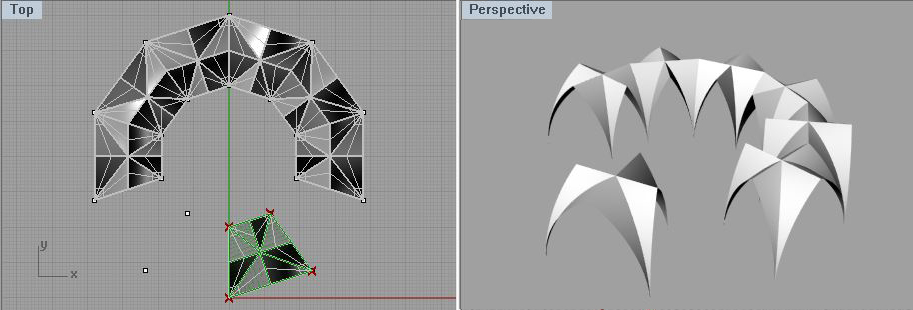COMPUTER AIDED ARCHITECTURAL DESIGN
Workshop
17 Notes, Weeks of November 24 and December 2, 2014

PYTHON SCRIPT COMPONENTS AND FUNCTIONS INSIDE GRASSHOPPER

These notes revisits techniques covered during October, and adds on the use of function definitions inside of Python components that we will cover in November.

1. Hello World and Simple Python Scripts

In some circumstances it may be more effective to use a Python script component in conjunction with other Grasshopper components. We begin with a few simple Python scripts. These first scripts are for introductory purposes and don't necessarily offer an advantage in terms of efficiency or clarity over other Grasshopper components. We will then move onto Python scripts which do offer advantages over other Grasshopper options in terms of conciseness and clarity of organization. Within these examples we take advantage of libraries and related function calls to perform some tasks, such as:

The above expression assigns to the variable "a" a line. The line generated by a procedure called "AddLine" that is located in "rhinoscriptsyntax" library abbreviated here as "rs". "AddLine" creates a line from two existing end points, which in the above example are named "point1" and "point2". More complete documentation on Python, examples, and associated libraries are located at the web site http://wiki.mcneel.com/developer/python. On the web site, the manual http://download.rhino3d.com/IronPython/5.0/RhinoPython101/ provides a comprehensive introduction and examples to establish a basic understanding . A few examples of the Python language are also presented here and developed separately.

To get started, launch Rhino and Grasshopper, and then within Grasshopper load the file helloWorld.gh.  The file shows two Python scripts, one that takes as input a text strings such as "Hello World!" and echoes it back, and the other that takes as input two numbers and outputs their sum.

In the case of "Hello World!", the Python examples follows a tradition of introducing programming languages that usually start with a function that prints or echos the words "Hello World!"  back to the user.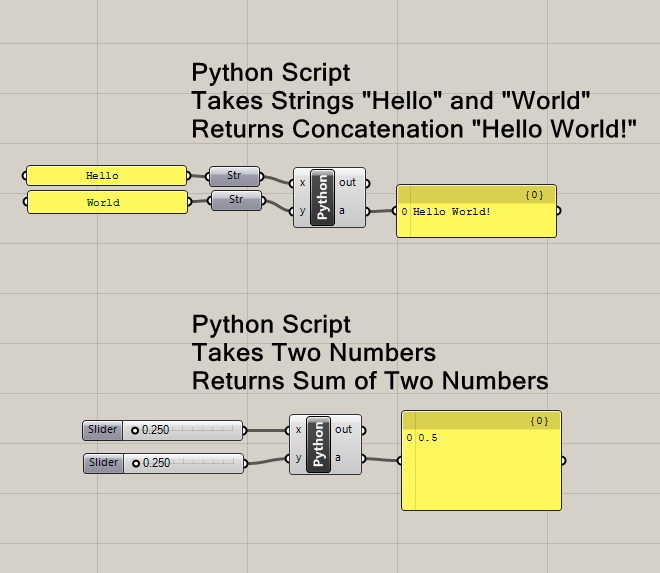Note in the above illustrated canvas window that the input text strings "Hello" and "World" appear in separate yellow text boxes. In turn the yellow text boxes are connected to the input port of two "Str" (string) Grasshopper components. The two "Str" components are in turn connected to the Python script input parameters "x" and "y".

To see the Python Script in detail, double click on "Python" icon in the Grasshopper window to launch the script editor.  The first line of Python script, as shown below, adds the two strings together and places an exclamation mark a the end. The same expression also assigns it to the output variable "a".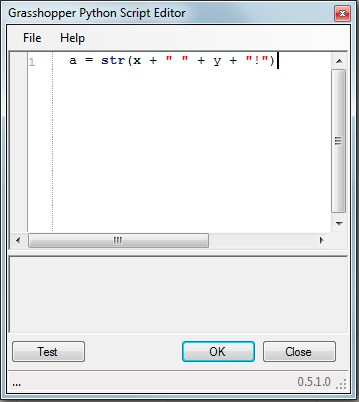The Python component in turn returns the parameter "a" through an output port into the input port of the yellow text box which is placed to the right of it in the canvas window as depicted below. The yellow text box echoes back the text string "Hello World!". However, if you modify either of the input text strings, then the output text string is modified accordingly, such as replacing the text string "World" with the text string "Virginia" in the example below.Note above that the two input arguments "x" and "y" are passed into the Python script component as strings ("Str"). The string designation  means that both "x" and "y" are can be assigned a text expression consisting of alphanumeric characters.

Note too that any change to the value of an input variable (such as "x" or "y")  inside the Python Script component  is limited in scope to be internal to the script function.  That is, the modification to the value of the variable internal to script function would not change its value outside the script.  Thus if inside the Python component we add the expression y = "virginia film festival". the value of y outside of the script would be unchanged from "Virginia".

Lets go into more detail regarding the Python component shown above. The expression in line 1 is:

a = str(x + " " + y + "!")

The statement assigns a string value to variable "a" by joining together four values:

1. the value of the string x,
2. a blank space " ",
3. the string y, and
4. the exclamation point "!".

Note that the use of the "+" symbol is used to add together (concatenate) the larger string from the four values "Hello", " ", World, "!", similar to how one might add several numbers together.
Once again, the variable "a" is also the output variable of the script. Thus, it in turn is directed to the input port of the yellow panel that displays the result "Hello World!".

Now we will reconstruct the script from scratch. Go to File/New Script. Go to the "Params" tab  and in the "Input" area, select the yellow panel.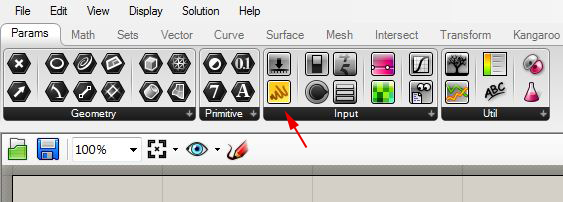Drag two yellow panels with the left-mouse button into the Grasshopper view window.Double click on each panel, and edit the text string  to become "Hello" on on panel and to become  "World" on  the other panel.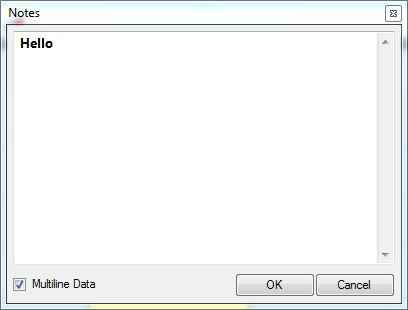The result is that the panels should transform as follows.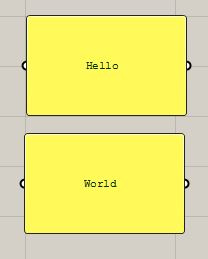Continuing with  the "Params" tab, go to the  "Primitive" area and select the string component symbolized by the letter "A".Drag two string components with the left-mouse button into the Grasshopper view window, and connect their input ports to the output ports of the two yellow panels.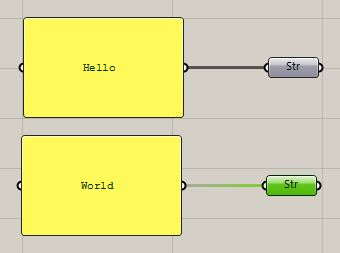Now go the the "Math" tab and in the "Input" area, select the blue and yellow Python component.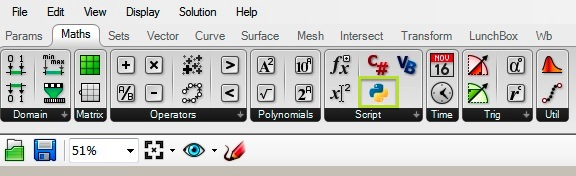Drag on Python component  with the left-mouse button into the Grasshopper view window and connect its input ports to the output ports of the two string components. Resize the yellow panels by moving the mouse over any of its edges, depressing the left-mouse button, and dragging the edge to the desired size.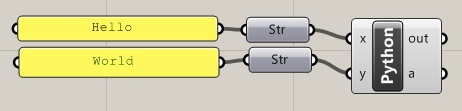Zoom into the Python component ,  right-click on the letter "x", go the the "Type hint" item, and change the type to "String". Do the same for the letter "y" variable.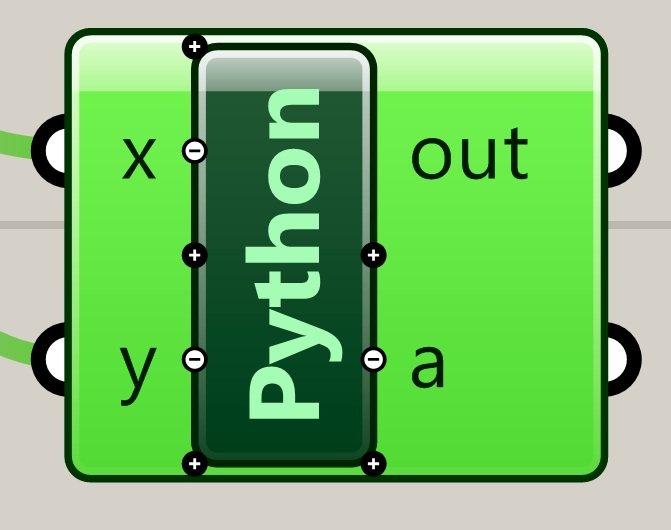Now, double-click on the Python component symbol in order to open it script editor. Note that it is essentially an emptry text file.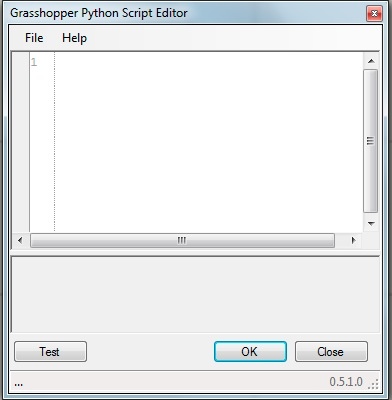Type in the single expression needed to complete the script.

a = str(x + " " + y + "!")Select the "OK" button on the lower right hand corner of the script editor to conclude editing the script. Go back to the "Params" tab and to the  "Input" area, select the yellow panel , add it to the right of the Python script within the Grasshopper view window.Connect the output port "a" of the Python script to the yellow panel to see the output.Note that it is generally quite useful to document the purpose and the input and output variables of the Python script with comment lines. Comment lines are not executed and are preceded by a number symbol # (e.g., #This is a comment line).   For example, double-click on the Python component, and add the comment lines:

#Returns "Hello World!
#INPUT: string x "hello" and y "world"
#OUTPUT: a concatenates x and y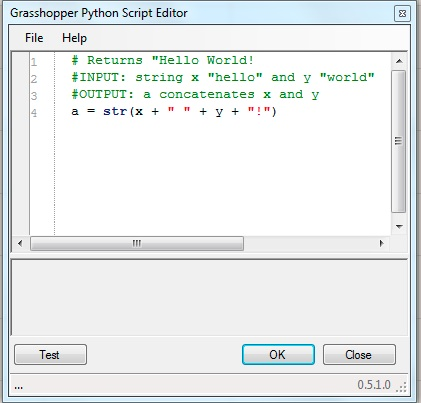The Python script to construct two numbers is constructed in a similar way. Go to the "Params" tab and from the "Input" and a number slider.Drag two number sliders into the Grasshopper view window. Now, similar to the "Hello World" example, go to the Math "Tab" and from the "Script" area drag Python component  with the left-mouse button into the Grasshopper view window and connect its input ports to the output ports of the two number slider components.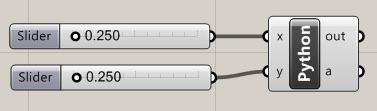Zoom into the Python component ,  right-click on the letter "x", go the the "Type hint" item, and change the type to "Double". Do the same for the letter "y" variable. Double click on the Python symbol in order to open the editor. Note once again that the editor
is essentially an empty file.The following comment lines and expressions are now added to complete the script and return the result in the variable a.

#INPUT: numbers x and y
#OUTPUT: sum of x and y

a = x + y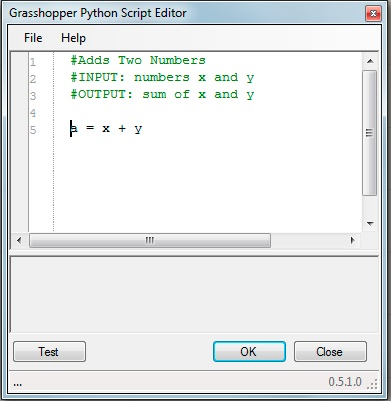Finally,
select the "OK" button on the lower right hand corner of the script editor to conclude editing the script. Go back to the "Params" tab and to the "Input" area, select the yellow panel , add it to the right of the Python script within the Grasshopper view window. Connect the output port "a" of the script to the input port of yellow panel to obtain the final result of the script.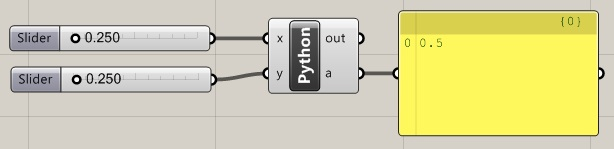2. Scripts to Draw a Few Graphic Primitives

Within the previous set of Grasshopper workshop notes (workshop 16), we covered how to generate a line from two points. Here we perform the same task by using a Python script to produce a grasshopper file makeSimpleLine.gh.

Initiate the script within Rhino by creating two points in the x, y ground plane.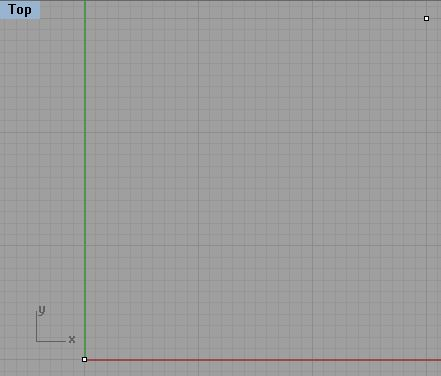Within Grasshopper, go to the "Params" tab and within the "Geometry" area, select and drag two point icons into the work area.Right-click on each of the two points and use the "set on point" option to connect them to the points created on the x, y plane.Next, similarly to "Hello World" example above, go the "Math" tab and within the "Script" area drag a "Python" component into the Grasshopper View window.Connect the the points to the " x"  and "y"  input ports of the Python script component. Zoom up to "x" variable, right-click on it and in the top line of the dialog box that follows, change the name to " point1".  Right click on "point1" again and change the Type hint option to "Point3d".  Similarly, rename the "y" variable to be "point2" and change the Type hint option to "Point3d"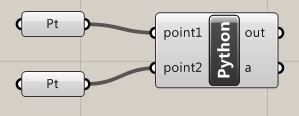Double-click on "Python" of the Python scripting component,  go to the script editor, and add the following expressions:

import rhinoscriptsyntax as rs
import Rhino as rc
import math

Add the following comment lines at the beginning of the script:

#Draws a linefrom two points
#sINPUTS: Two points
#OUTPUT: A line determined by the two points

Add the following statements after the comment lines:

Within the script editor we see the following result:This completes the script and resulting line should now appear inside Rhino.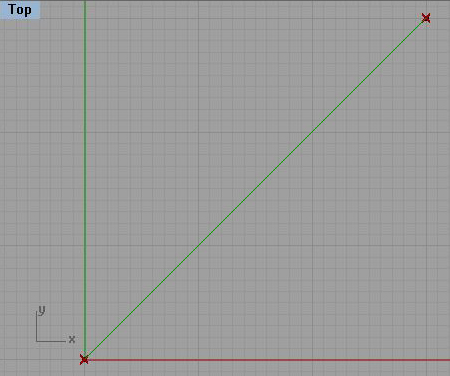Move either of the two points and the line is modified accordingly.The same script can be modified to draw a rectangle, makeSimpleRectangle.gh,  from the initial end points.

First, note that we can extract the coordinates x1, y1, z1 from point1 and the coordinate x2, y2, and z2 from point2. We can combine the two sets of coordinate  to get the four points cornerPt1, cornerPt2, cornerPt3 and cornerPt4, that  detemine the rectangle. Assuming that both point1 and point2 are in the x-y plane, also note that z1 = z2 = 0. That is, we have the following way to establish the values of the four corner points.

cornerPt1 - based upon  x1, y1, z1
cornerPt2 - based upon x2, y1,  z1
cornerPt3 - based upon x2, y2,  z1
cornerPt4 - based upon x1, y2,  z1.

With this in mind, we can edit the Python script as indicated below to get a rectangle. Note that comment lines added to the script describe what is achieved by each step.

#'Draws a rectangle from two points
#'INPUTS: Two points
#OUTPUT: A rectangle (polyline) determined by the two points

#Create the corner points from the x, y, and z coordinates of point1 and point2
cpt1 = rc.Geometry.Point3d(point1.X, point1.Y, point1.Z)
cpt2 = rc.Geometry.Point3d(point2.X, point1.Y, point1.Z)
cpt3 = rc.Geometry.Point3d(point2.X, point2.Y, point1.Z)
cpt4 = rc.Geometry.Point3d(point1.X, point2.Y, point1.Z)

#Add the first corner point from the x, y, and z coordinates of point1 to complete the polyline
cpt5 = rc.Geometry.Point3d(point1.X, point1.Y, point1.Z)

#Create the polyline from the list of points
line1 = rs.AddPolyline([cpt1, cpt2, cpt3, cpt4, cpt5])

#Return the polyline from the Python script
a = line1

The script editor is now modified as follows.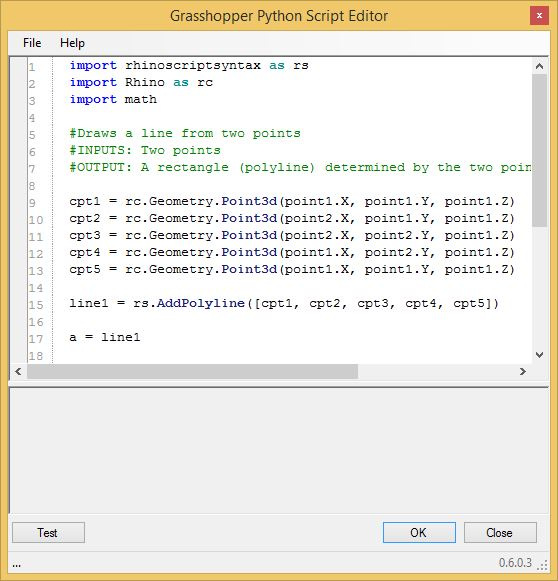The script as now completed draws a rectangle. Note that moving the lower left corner point from within Rhino dynamically redraws the rectangle.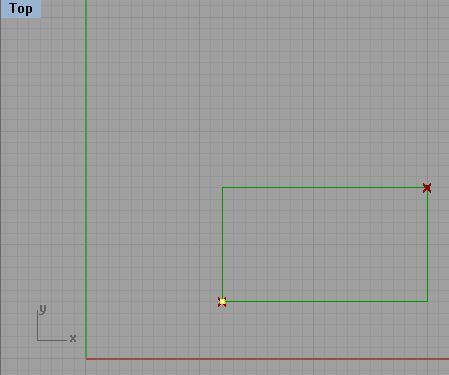Looking at this script in greater detail, observe that we have embedded a point list [cpt1, cpt2, cpt3, cpt4, cpt5] withing the statement

line1 = rs.AddPolyline([cpt1, cpt2, cpt3, cpt4, cpt5])

We add the  lower-left point to both the beginning and the end of the list to ensure that we get a closed polyline..

Note too that we introduced a polyline object variable with the statement line1 = rs.AddPolyline

The polyline object line1 is thus initialized with the listing of points.

3. Adding Functions to a Python Script

Adding functions can help to bring clearer organization, modularity and at times greater efficiency to a Python component. Functions are set apart with a "def" expression. For example, we can remake the above rectangle Python component into a Grasshopper script makeSimpleRectangleWithFunction.gh with a "def makeRect(point1, point2)" function:Notes:

3.1. The function has two arguments, "point1" and "point2". It is called with the expression:

a = makeRec(point1, point2).

3.2. The function definition "def makeRec(point1, point2): ..." must be placed in the Python text in the lines above where it is called. That is, it is defined in lines 9 thorugh 16 of the script editor. It is then callled in line 19 of the script editor with the expression "a = makeRec(point1, point2)". In Python in general the function must be defined before it can be called.

3.3. Line #15 of the "makeRec" function assigns  the polyline rectangle to the vaiable "rect".

3.4. The last line #16 of the "makeRec" function returns the polyline rectangle with the expression "return rect".

3.5. In line #19 of the Python compnent the rectangle that is output from the "makeRec" function in turn is assigned to the Python component output variable "a".

3.6. The rectangle assigned to output variable "a" in turn is displayed Rhino drawing window.

5. Koch Curve with Recursive Function

The Grasshopper file kochCurve.gh contains a Python component can be used to draw a Koch curve based upon an equilateral triangle in a corresponding Rhino file.

Connect the "Crv" input component in the Grasshopper canvas to three lines of an equilateral triangle. That is, right click on the "crv" component,  and in the pop-up dialog that follows, pick the "set multple curves" option, and select the three lines of the equilateral triangle. Once slected the lines of the equilaterial triangle will highlight in green, and the Koch curve will be generated in this case with a recursive level "r" set to 4.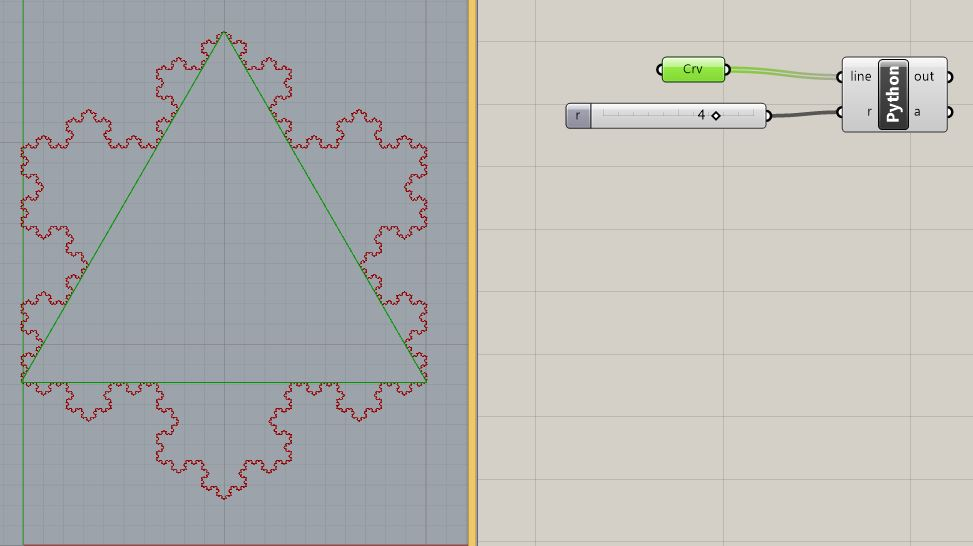Now select the python script component in the Grasshopper canvas window and the Koch curve itself will highlight in a green color.Double-click on the Python component to open the Python Script Editor. A function named "recursiveLine" in the Python component handles the recursive self-similar fractal. The first part of the function marks off each line with points that subdivide it into thirds. It then creates a polyline of four line segments.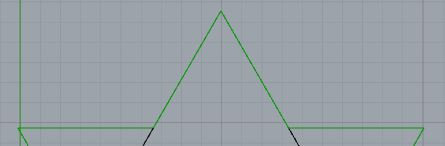This is accomplieshed by lines 14 through 27 in the Python script below.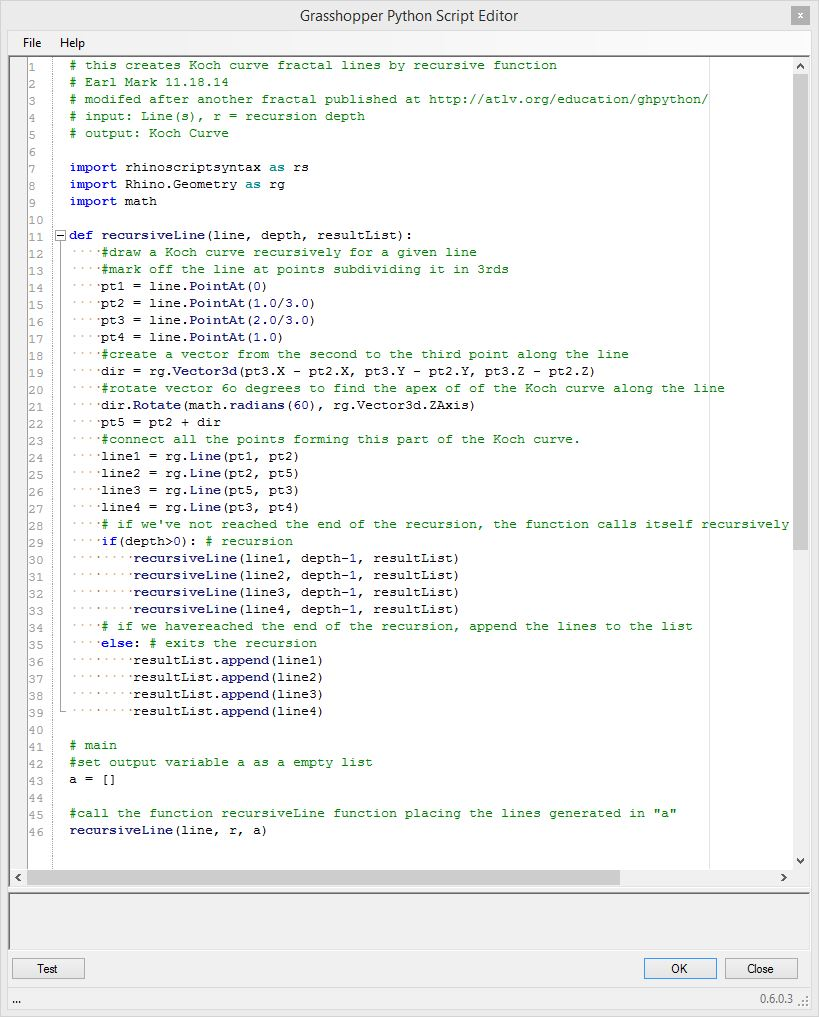The function "recursiveLine" is recursive in that it continues to call itself repeatedly until reaching an end condition where "depth", the integer number of recursive calls to itself, counts down to "0". Note in paritcular the "if else" conditional statement included within the function in lines 28 through 39 in the Pythion Script:

if(depth>0): # recursion
........recursiveLine(line1, depth -1, resultList)
........recursiveLine(line2, depth -1, resultList)
........recursiveLine(line3, depth -1, resultList)
........recursiveLine(line4, depth -1, resultList)
else: # final depth
........resultList.append(line1)
........resultList.append(line2)
........resultList.append(line3)
........resultList.append(line4)

Within the "if else" statement, if depth is greater than 0, the the "if" part of the statement is executed,  the function calls itself, and  the  value of depth is decremented by -1. However, if the value of depth decrements to the point where it is not  greater than 0, then the "else" portion of the statement is executed, and the final line is appended to a list of lines assigned to "resultList".

Note also that the function "recursiveLine" is defined within the Python script in lines 11 through 39 before it is called in line 46. That is, within the Python language, the function must exist before it can be used. The program is really activated on line 43. The comment lines 41 and 42 are displayed in green are are not executed:

# main
# set output variable as an empty list

a = [ ]

In the expression a = [ ] the output varable "a" is created as an empty list:

Next, in line 46 of the Python script, the function "recursiveLine" is called with three input variables:

recursiveLine(line, r, a)

The variables are:

line  - the three lines of the equalateral triangle input from rhino,
r - the number of recursions
a - the empty list in which the recursively generated lines will be place.

Note that the variable "a'" is listed third in line 46 above. However, in the the definition of the function itself, in line 11 of the script editor, the third argument is "resultList".

def recursiveLine(line, depth, resultList)

Here, the variable "a" fills the slot occupied in the function definition by the variable "resultList". The value of "a" is therefore used to initiate the value of "resultList". Moreover, the lines generated within the function are placed in "resultList", and they are in turn placed back into the output  variable "a".

(Programmers with more experience may be familiar with the concept of passing variables "by value" or "by reference". Typically, Python passes variables "by value". Thus in this example of the program "recursiveLline", the modificaiton to the variable "recursiveList" wouldn't normally impact the value of the variable "a". However, in this implementation of Python, the value of "a" is directly modified as if it were passed "by reference".)

6. Fractal Tree with Recursive Function

The grasshopper file branches.gh containing a Python component can be used to draw a fractal tree based upon a point or a series of points in a Rhino file such as trees.3dm. This function is recursive in that it continues to call itself repeatedly until reaching an end condition where "n", the number of recursive calls to itself, counts down an integer number to "1".

To generate two trees, right-click on the "Pt"  input parameter, pick the "set mulitple points" option, and pick two points in the ground plane of the Rhino File (marked in Green in the "Top"  view below). The Ptyhon script generates the fractal trees for each point as more clearly evident in the "Front" view below.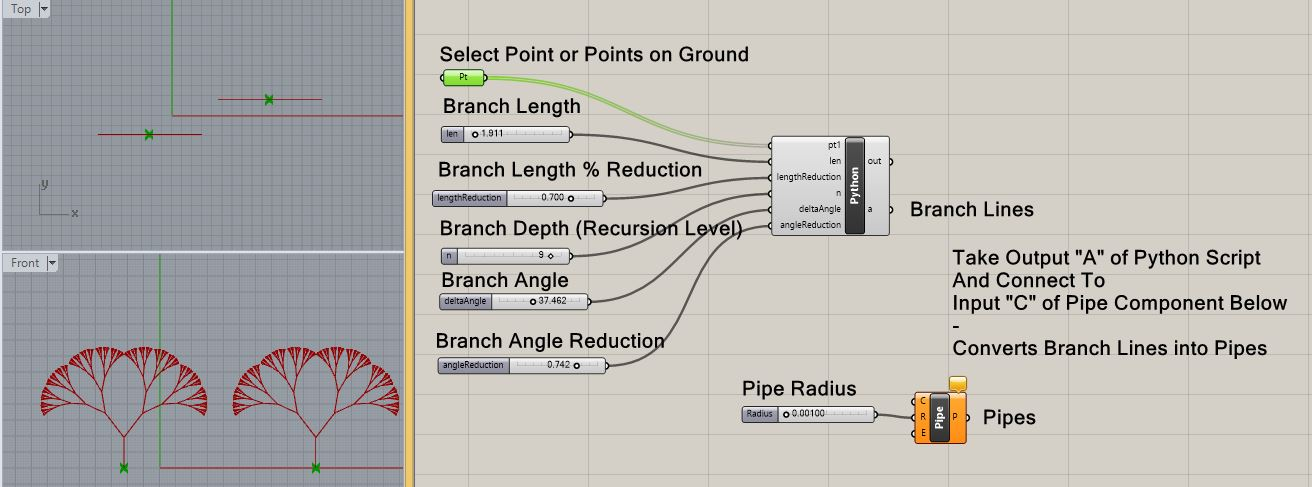The Python script itself goes recursively into a left branch and a right branch for each level of recursion. Here are three separate versions of the tree where the recursion leves is set  3, 4, 7 and 10 respectively: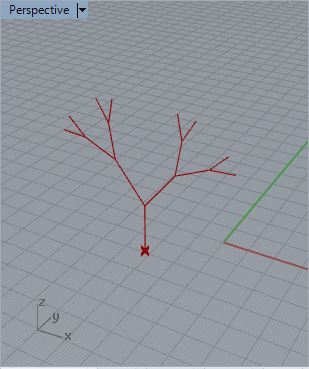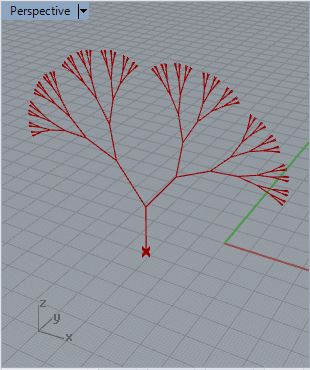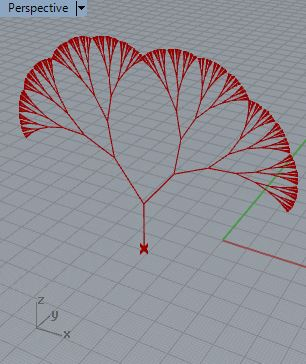Note that by connecting the output "a'' of the Python component to the input port "C" of the "Pipe" component,  the branches of the tree, which are represented as lines,  are converted into cylindrical surfaces with a radius of 0.02250.  Here for example we see such surfaces generated for recursion level set to 6.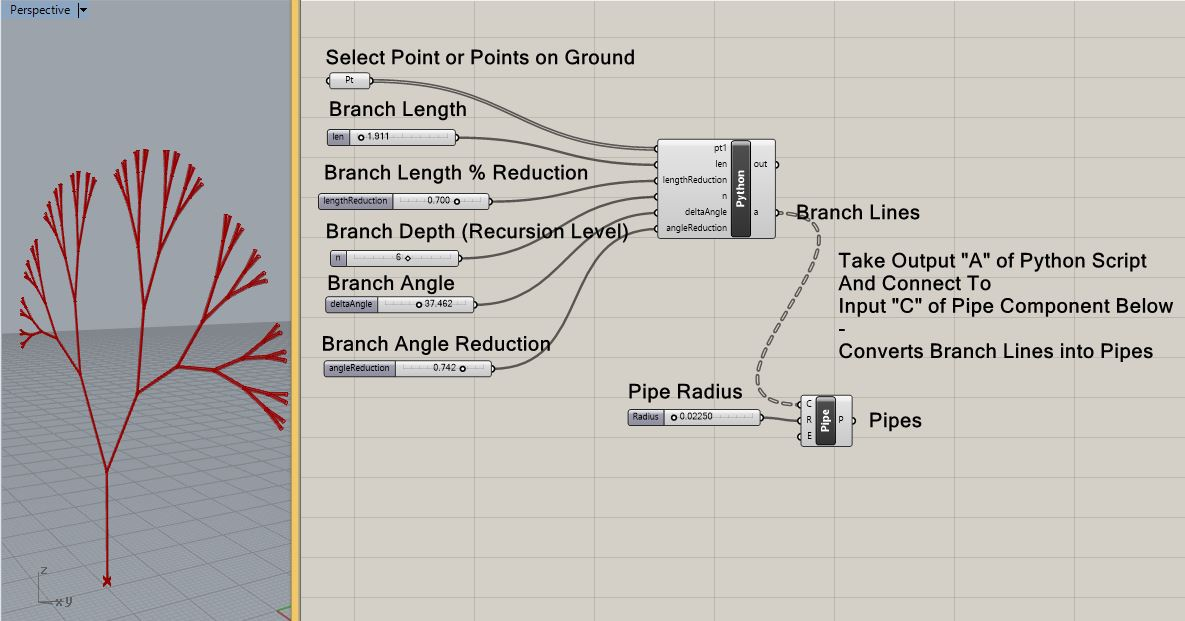For more details on the recursive steps, view the comment lines directly in the Python component.

7. Script That Uses Iteration to Express Recurring Relationship

To draw a series of inwardly rotating polylines in the Grasshopper file makeInwardlyTurningRectangle.gh starts with an outer rectangle. It continues with each new rectangle in the series stepping upward in elevation. In this example we repeat a similar operation (i.e., rotating and elevating a new rectangle) a specified number of times. Note that here we see the script without the user defined functions. In the next example, we will revisit the same code rewritten with such internal functions.Note that in the figure above, each inner polyline is calculated from point that is 40% along along each of the four  sides of the next outer polyline. In the Python script used to generate this figure, a technique referred to as iteration is used to repeat the same process a given number of times. A number of such iterative techniques exist within the Python scripting language. Within the current example, we use a "while" expression that loops continuously through a series of indented expressions during the time that some integer " i"  increments in value from 1 to the number times we  we want to repeat loop. In "pseudo-code" (not Python actual code, but more general words), the "while" statement has the following format :

while (i <= iterations) :
....Create a new polyline from the four points
.... Add the polyline to a list of polylines
....Calculate four points of each new polyline

Note that the "while" statement only  includes the indented statements which follow it.

First, lets begin by adding to new parameters to the Grasshopper Script from Part 3. Go to the "Params" tab and in the "Input" area add two numerical sliders to the Grasshopper view window.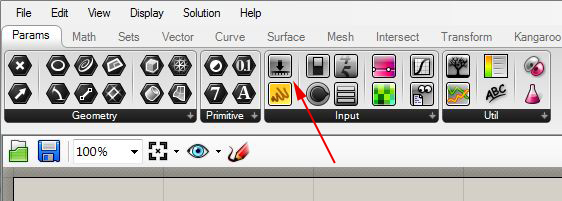Double-click on the word "slider" and rename the numerical sliders iterations,  param Dist, and  stepSize. Adjust the iterations slider so that it ranges in value from 0 to 20, and adjust the type to"N" (integer numbers).The paramDist slider by default ranges in value from 0.0 to 1.0, which we can use to represent 0% to 100% of the distance along any side of the last outer polygon. Similarly, the stepSize slider by default ranges in value from 0.0 to 1.0, which we can use to represent the elevation change of each inner rectangle above the outer rectangle that preceded it..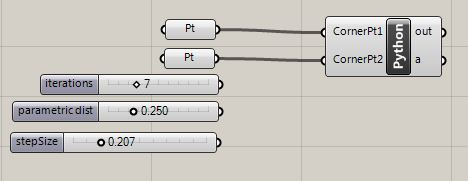Zoom up on the  Python script symbol until "+" symbols appear below the input variables "point1" and "point2".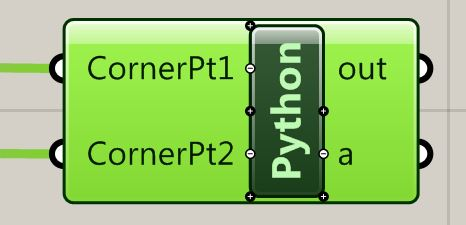Select the "+" symbol below  "point2" to add a variable "paramDist".  Change the default name of "x" to "paramDist" and change the Type hint option to "Double". Similarly, select the "+" symbol below  "paramDist".  Change the default name of "x" to "numTimes" and change the Type hint option to "Integer".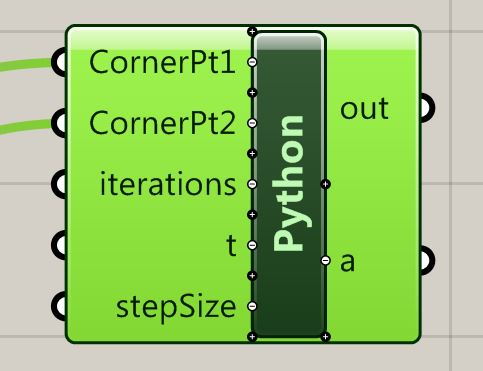Next, connect the output ports of the two numerical sliders to the new input variables of the Python script.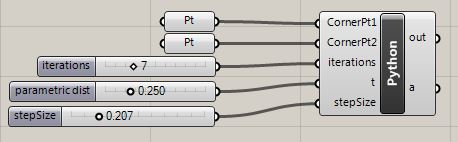Now, to create the series of rectangles we edit the Python script to incorporate the following text. Comment lines preceded by a # describe what each step of the Python script does.

import rhinoscriptsyntax as rs
import Rhino as rc
import math
#Draws a series of rotating rectangles from two points
#INPUTS: Two points
#OUTPUT: A line determined by the two points

#initiate a polyline list
lines = [ ]

#derive x,y,z values of four corner points from two input points
x1 = CornerPt1.X
y1 = CornerPt1.Y
z1 = CornerPt1.Z

x2 = CornerPt2.X
y2 = CornerPt1.Y
z2 = CornerPt1.Z

x3 = CornerPt2.X
y3 = CornerPt2.Y
z3 = CornerPt2.Z

x4 = CornerPt1.X
y4 = CornerPt2.Y
z4 = CornerPt2.Z

#initiate four corner points
cpt1 = rc.Geometry.Point3d(x1, y1, z1)
cpt2 = rc.Geometry.Point3d(x2, y2, z2)
cpt3 = rc.Geometry.Point3d(x3, y3, z3)
cpt4 = rc.Geometry.Point3d(x4, y4, z4)
cpt5 = rc.Geometry.Point3d(x1, y1, z1)

#initiate counter
i = 0

while (i < iterations):
....#create a polyline from the four corner points
.... line1 = rs.AddPolyline([cpt1, cpt2, cpt3, cpt4, cpt5])
....
.... #append the polyline to the polyline list
....lines.append(line1)
....
....#get x, y, z values of interior points along each segement of the polyline
....xm1 = cpt1.X + (cpt2.X - cpt1.X) * t
....ym1 = cpt1.Y + (cpt2.Y - cpt1.Y) * t
....zm1 = stepSize + cpt1.Z + (cpt2.Z - cpt1.Z) * t
.... m2 = cpt2.X + (cpt3.X - cpt2.X) * t
....ym2 = cpt2.Y + (cpt3.Y - cpt2.Y) * t
....zm2 = stepSize + cpt2.Z + (cpt3.Z - cpt2.Z) * t
....xm3 = cpt3.X + (cpt4.X - cpt3.X) * t
....ym3 = cpt3.Y + (cpt4.Y - cpt3.Y) * t
....zm3 = stepSize + cpt3.Z + (cpt4.Z - cpt3.Z) * t
....xm4 = cpt4.X + (cpt1.X - cpt4.X) * t
....ym4 = cpt4.Y + (cpt1.Y - cpt4.Y) * t
....zm4 = stepSize + cpt4.Z + (cpt1.Z - cpt4.Z) * t
....
....#reset corner points to the new set of points
....cpt1 = rc.Geometry.Point3d(xm1, ym1, zm1)
....cpt2 = rc.Geometry.Point3d(xm2, ym2, zm2)
....cpt3 = rc.Geometry.Point3d(xm3, ym3, zm3)
....cpt4 = rc.Geometry.Point3d(xm4, ym4, zm4)
....cpt5 = rc.Geometry.Point3d(xm1, ym1, zm1)
....
....i = i + 1

#return the list of polylines from the Python script
a = lines

The  Script Editor now appears as follows.The set of polylines output of the Python script can be input onto a surfaces component in order to convert the series of rotating rectangles into a seres of rotating surfaces.  Go to the "Surface" tab in grasshopper, and select the "Boundary Surfaces" component.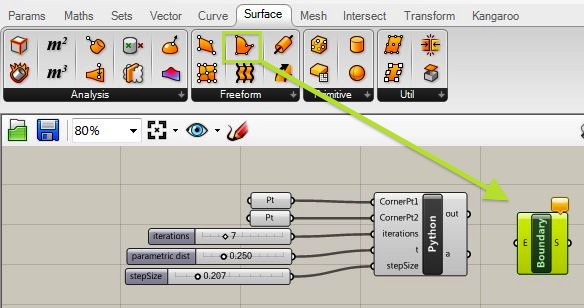Connect the output port  "a" to the input port "b" of the bounary surface conmponent to generate the series of rotating surfaces.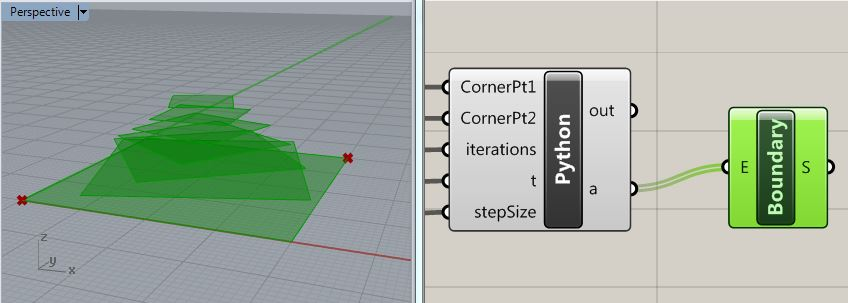8. Rotating Rectangle Script Redeveloped with Functions

The grasshopper file makeTurningRectangleWithFunctions.gh revisits the turning rectangle script, but incorporates function definitions to improve modularity and clarity of organzation.

9. Script That Uses Iteration to Generate A Helix

To draw a series of inwardly rotating polylines form the outer rectangle, we needed to repeat a similar operation a specified number of times. Similar to the rotating figure above, a helix, such as determined by, adjustableHelixPython.gh, can also be described by a loop. The vertices that are used to define the spiral are also created in a repeated operation for a specified number of times.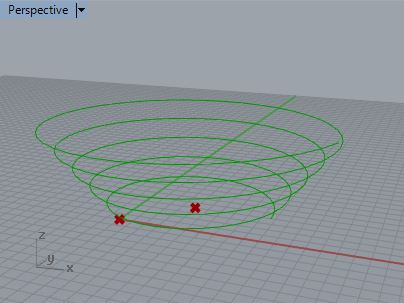Using tools described previously, initiate the helix by placing two point parameters with a Grasshopper window, and by also adding four numerical slider parameters. One point will be used to identify the center of the spiral. The second point will be used to define the radius. The following sliders should have the following value types and ranges:

 Name of Slider Description Type Min Value Max Value numRev number of revolutions in the spiral Integer (N) 1 25 numSide number of sides in each revolution of the sprial Integer (N) 3 360 heightIncrement height increment in each revolution of the spiral Floating Point (R) 0 25 radiaIncrement radius increment in each revolution of the sprial Floating Poing(R) -25 25

Next, connect the points in  Rhino  to the point input parameters, as done in previous examples. The two points will serve as the center point and radial point of the sprial.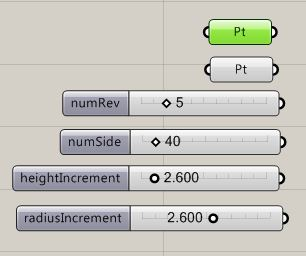Now, add a Python script component to the Grasshopper View window and add the following input variables and types (use the Type hint feature as done in earlier examples):

 Name of Variable Description Type hint centerPt center point of helix Point3d radiusPt radial point of helix Point3d numRev number of revolutions in the spiral int numSide number of sides in each revolution of the sprial int heightIncrement height increment in each revolution of the spiral float radiaIncrement radius increment in each revolution of the sprial float

Note that the sliders for numRev and numSide ares specified at int (integers), but that the input variables numRev and numSide are specified as float (i.e. floating point numbers). Thus the values are converted from integers to floating point numbers when connected to the Python script. The integer designation for the sliders ensures that only whole numbers are selected. This conversion to floating point numbers ensures that floating point accuracy is maintained in the Python script where needed in some operations.Finally, double-click on the Python script and add the following expression into the script editor.

import rhinoscriptsyntax as rs
import Rhino as rc
import math
#draws a helix
#INPUTS:
#numRev = number of revolutions in helix
#numSide = number of sides per revolution
#heightIncrement = height increment per revolution
#OUTPUT:
#a = pline = polyline approximation of helix curve

#Declare variables for spiral point list, vector from center pt to radius pt, and radius
ptList = []

#Declare variable to hold incremental values of rotation angle, height and radius for each point in the spiral
angleIncrement = 360.0/numSide
ptHeightIncrement = heightIncrement/numSide

#Declare variable to hold the initial values for the angle, height and radius
curAngle = 0
curHeight = 0

#Loop to generate points in the spiral
i = 1 #initiate counter for loop
while ( i < numRev * numSide) :
....z = centerPt.Z + curHeight
....#Add each successive point to the point list
.... ptList.append(curPt)
....
....#Advance the values of the current angle, current height and current radius for the next point in the spiral
....curAngle = curAngle + angleIncrement
....curHeight = curHeight + ptHeightIncrement
....i = i + 1 #increment loop counter

#Determine a polyline from the point list

#Return the polyline to Rhino
a = pline

Select the "OK" button in the editor and the Grasshopper file will produce the helix as depicted in the following image.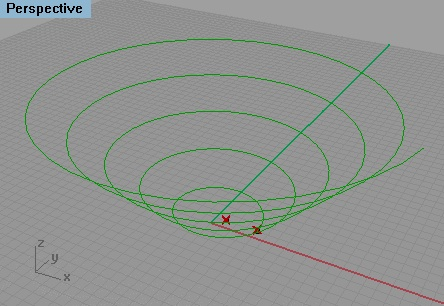10. Script That Uses Iteration to Generate A Ramp

The helix script can be enhanced to become todraw two spirals that form a ramp, as illustrated by the Grasshopper file adjustableRampPython.gh.The essential strategy for looping through vertices is the same. Begin by adding a radial point within Rhino to determine the location of the second helix.Next, within Grasshopper add a new point input parameter radialPt2 and connect it to the recently added radial point, and a corresponding Point3D variable "radiusPt2" to the Python script.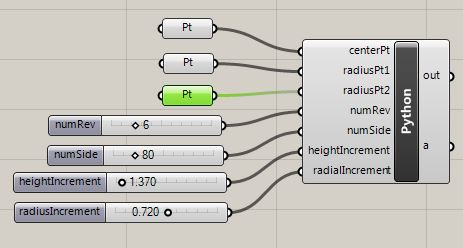Within the Python script, we now double up on the points so as to produce two spirals rather than one spiral. Furthermore, rather than use the points to form a polyline as in the previous example of the helix, we use them to form two bspline curves. Next, the bspline curves are used to generate a ruled surface. Whereas polylines can not be used to generate a ruled surface, the bspline curves can be used to generate one.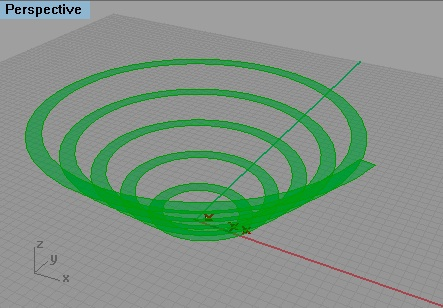The Python scrip is modified as follows. See the comment lines below for details:

import rhinoscriptsyntax as rs
import Rhino as rc
import math
#draws a helix
#INPUTS:
#numRev = number of revolutions in helix
#numSide = number of sides per revolution
#heightIncrement = height increment per revolution
#OUTPUT:
#a = pline = polyline approximation of helix curve

#Declare variables for spiral point list, vector from center pt to radius pt, and radius
ptList = []
ptList2 = []

#Declare variable to hold incremental values of rotation angle, height and radius for each point in the spiral
angleIncrement = 360.0/numSide
ptHeightIncrement = heightIncrement/numSide

#Declare variable to hold the initial values for the angle, height and radius
curAngle = 0
curHeight = 0

#Loop to generate points in the spirals
i = 1 #initiate counter for loop
while ( i < numRev * numSide) :
....z = centerPt.Z + curHeight
....z2 = centerPt.Z + curHeight
....
....#Add each successive point to the point list
....ptList.append(curPt)
....ptList2.append(curPt2)
....
....#Advance the values of the current angle, current height and current radius for the next point in the spiral
....curAngle = curAngle + angleIncrement
....curHeight = curHeight + ptHeightIncrement
....i = i + 1 #increment loop counter

#Determine a polyline from the point list

#Build lofted surface between two curves

a = rampSurf

Note that we used some new statements to determine a surface.

fvv#Build lofted surface between two curves

In particular we used a function library "rs.AddLoftSrf". A number of functions have been created for Grasshopper to generate geometry and handle other requirements.  The current listing of functions and related examples can be found in the web site: http://wiki.mcneel.com/developer/python

11. Python Scripts That Include Functions

This example, makeQuadripartiteVaultPython.gh,  that generates a quadripartite vault from four corner points on the x-y ground plane. It uses functions to help modularize the code.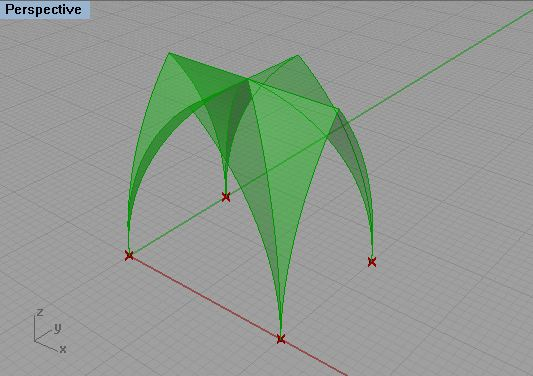The basic components of the script are the same as what was used in previous examples for this set of workshop notes. Four "pt" components are linked to the four points on the ground in counter-clockwise order. A numerical slider that ranges in value from -5 to 5 is used to determine the height difference if any between the central crossing arches and the side archest of the vault. The Python script accepts the four ground points and height difference number as inputs. It returns the vault surfaces as output.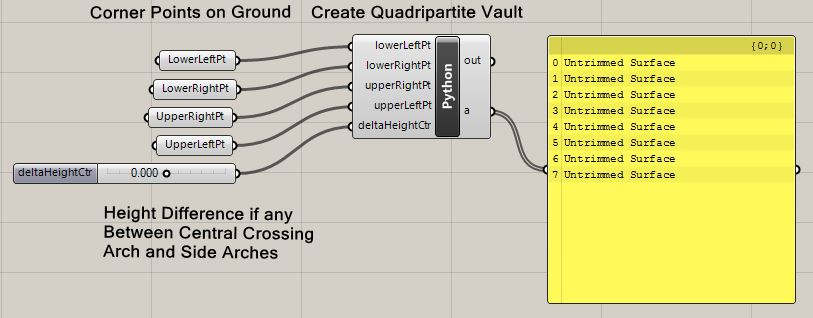Note that on the right-hand side of the Grasshopper window, a yellow panel is used to echo back the output of the function. To place such as yellow panel inside the Grasshopper window,  go to the "Params Tab"and the "Input Area", select a yellow panel and connect to the output of the Python script

Within the Python script  There are  "def"  functions to perform more of the explicit tasks called upon by the main body of code. In this example, the construction of the quadripartite vault follows a seven step sequence of:

1. Start with the four corner points on the ground in counter-clockwise order (lower-left, lower-right, upper-right, upper-left)
2. Determine the center of the vault on the ground
3. Determine the center arches apex point
4. Determine the side arches apex points
5. Determine the central crossing arches
6. Determine the side crossing arches
7. Loft vault surfaces between corresponding arches

The main body of code meta logic of what is done at an abstract level. Note too that all the "def" statements must precede the main body of code which relies upon them. The is due to the fact the Python scripts are compiled at runtime, a detail beyond the scope of the workshop.

import rhinoscriptsyntax as rs
import Rhino as rc
import math

#Creates A Quadripartite Vault from four points on the the Ground (x-y plane)
#INPUTS: corner points on ground LlowerLeftPt, lowerRightPt, upperRightPt, upperLeftPt
#deltaHeightCtr = difference betweend apex height of central crossing arch and apex of side arches
#OUTPUTS: Surflist = list of eight quadripartite surfaces

def GetCentroid(lowerLeftPt, lowerRightPt, upperRightPt, upperLeftPt):
....#Determine centroid of four point
....x = (lowerLeftPt.X + lowerRightPt.X + upperRightPt.X + upperLeftPt.X) / 4.0
....y = (lowerLeftPt.Y + lowerRightPt.Y + upperRightPt.Y + upperLeftPt.Y) / 4.0
....z = (lowerLeftPt.Z + lowerRightPt.Z + upperRightPt.Z + upperLeftPt.Z) / 4.0
....centroidPt = rc.Geometry.Point3d(x, y, z)
....return centroidPt

def GetCtrApexPt(lowerLeftPt,lowerRightPt,upperRightPt,upperLeftPt,CentroidPt):
....#Determine central apex point based upon corner point of vault, centroid on ground and CtrApexPt
....#Determine vectors between opposite corner points on ground and get their respective lengths
....vect1 = rs.VectorCreate(upperRightPt, lowerLeftPt)
....dist1 = rs.VectorLength(vect1)
....vect2 = rs.VectorCreate(upperLeftPt, lowerRightPt)
....dist2 = rs.VectorLength(vect1)
....#Determine the radius of the central cross arches based upon the average distance between opposite ground corner points
....radius = (dist1 + dist2) / 4.0
....CtrApexPt = rc.Geometry.Point3d(CentroidPt.X, CentroidPt.Y, CentroidPt.Z + radius)
....return CtrApexPt

def GetSideApexPt(EndPoint1, EndPoint2, CtrApexPt, deltaHeightCtr):
....#Determine side apex point based upon springing points of side arches, central apex point, and height difference if any with central apex point
....midPt = rc.Geometry.Point3d((EndPoint1.X + EndPoint2.X) / 2.0, (EndPoint1.Y + EndPoint2.Y) / 2.0, (EndPoint1.Z + EndPoint2.Z) / 2.0)
....SideApexPt = rc.Geometry.Point3d(midPt.X, midPt.Y, midPt.Z + CtrApexPt.Z + deltaHeightCtr)
....return SideApexPt

def MakeArcs(EndPoint1, ApexPt, EndPoint2):
....#Determine crossing arch halves CrossArcA and CrossArcB
....#Determine up vector tangent to arc at springing point
....UpVec = rc.Geometry.Vector3d(0,0,1)
....#Determine arches from endpoints and tangent vector
....Arc1 = rc.Geometry.Arc(EndPoint1, UpVec, ApexPt)
.... Arc2 = rc.Geometry.Arc(EndPoint2, UpVec, ApexPt)
....#Convert arc to arc-curve for compatibility with loft tool
....Arcc1 = rc.Geometry.ArcCurve(Arc1)
....Arcc2 = rc.Geometry.ArcCurve(Arc2)
....arcs = []
....arcs.append(Arcc1)
....arcs.append(Arcc2)
....return arcs

def GenVaultSurf(Arc1, Arc2):
....#Loft surface between corresponding arccurves
....#return first element of vaultSurf list - the actual surface generated
return vaultSurf

#Declare list variable for all surfaces
SurfList = [ ]

#Get the center of the vault on the ground
CentroidPt = GetCentroid(lowerLeftPt, lowerRightPt, upperRightPt, upperLeftPt)

#Determine the center arches apex point
CtrApexPt = GetCtrApexPt(lowerLeftPt, lowerRightPt, upperRightPt, upperLeftPt, CentroidPt)

#Determine each side arch apex point point
SideApexPt1 = GetSideApexPt(lowerLeftPt, lowerRightPt, CtrApexPt, deltaHeightCtr)
SideApexPt2 = GetSideApexPt(lowerRightPt, upperRightPt, CtrApexPt, deltaHeightCtr)
SideApexPt3 = GetSideApexPt(upperRightPt, upperLeftPt, CtrApexPt, deltaHeightCtr)
SideApexPt4 = GetSideApexPt(upperLeftPt, lowerLeftPt, CtrApexPt, deltaHeightCtr)

#Determine the central crossing arch halves
arcList1 = MakeArcs(lowerLeftPt, CtrApexPt, upperRightPt)
CrossArc1a = arcList1
CrossArc1b = arcList1
arcList2 = MakeArcs(upperLeftPt, CtrApexPt, lowerRightPt)
CrossArc2a = arcList2
CrossArc2b = arcList2

#Determine the side arch halves
arcList3 = MakeArcs(lowerLeftPt, SideApexPt1, lowerRightPt)
EndArc1a = arcList3
EndArc1b = arcList3
arcList4 = MakeArcs(lowerRightPt, SideApexPt2, upperRightPt)
EndArc2a = arcList4
EndArc2b = arcList4
arcList5 = MakeArcs(upperRightPt, SideApexPt3, upperLeftPt)
EndArc3a = arcList5
EndArc3b = arcList5
arcList6 = MakeArcs(upperLeftPt, SideApexPt4, lowerLeftPt)
EndArc4a = arcList6
EndArc4b = arcList6

#Loft surfaces between corresponding side-arch half and central crossing arch-half and add to SurfList
vaultSurf = GenVaultSurf(EndArc1a, CrossArc1a)
SurfList.append(vaultSurf)
vaultSurf = GenVaultSurf(EndArc1b, CrossArc2b)
SurfList.append(vaultSurf)
vaultSurf = GenVaultSurf(EndArc2a, CrossArc2b)
SurfList.append(vaultSurf)
vaultSurf = GenVaultSurf(EndArc2b, CrossArc1b)
SurfList.append(vaultSurf)
vaultSurf = GenVaultSurf(EndArc3a, CrossArc1b)
SurfList.append(vaultSurf)
vaultSurf = GenVaultSurf(EndArc3b, CrossArc2a)
SurfList.append(vaultSurf)
vaultSurf = GenVaultSurf(EndArc4a, CrossArc2a)
SurfList.append(vaultSurf)
vaultSurf = GenVaultSurf(EndArc4b, CrossArc1a)
SurfList.append(vaultSurf)

a = SurfList

nd Sub

Sub functions are defined to:

1. GetCentroid: Finds the centroid of four points.
2. GetCtrApexPt: Find the central apex point of the vault.
3. GetSideApexPt: Find the apex point of the side arches relative to the central apex point.
4. MakeArcs: Make arcs for each of the side and central crossing arches
5. GenVaultSurf: Generate vault surfaces between each corresponding side-arch half and central crossing arch half.

Note that each of the sub functions (defined forllowing  the key word "def")  has input data passed to them by means of variables, and returns data by means of a return statement, similar how the main body of the script has input data passed to it and returns data.

The main body of the script is somewhat like a general contractor that oversees overall workflow, and indeed can be conceived of without being focused on the details. The sub functions are like sub-contractors and employed multiple times within the overall process where needed. Given how the geometry has been defined, it is possible to move any of the ground points and determine a more asymmetrical vault.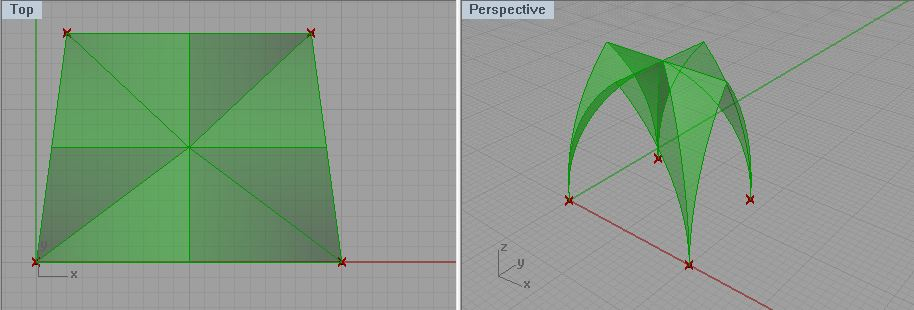Or, by entering a series of points, it is possible to replicate the arrangement of a nave, crossing, or ambulatory.